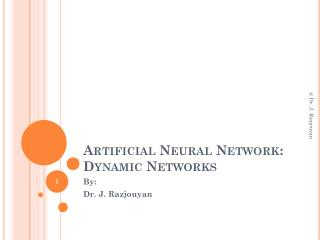DownloadDownload PresentationArtificial Neural Network: Dynamic Networks

# Artificial Neural Network: Dynamic Networks

Download Presentation## Artificial Neural Network: Dynamic Networks

- - - - - - - - - - - - - - - - - - - - - - - - - - - E N D - - - - - - - - - - - - - - - - - - - - - - - - - - -
##### Presentation Transcript

1. Artificial Neural Network:Dynamic Networks @ Dr. J. Razjouyan By: Dr. J. Razjouyan

2. Introduction • NN classified into : • Static : • Static (feedforward) networks have no feedback elements and contain no delays; the output is calculated directly from the input through feedforward connections. • Dynamic : • the output depends not only on the current input to the network, but also on the current or previous inputs, outputs, or states of the network. @ Dr. J. Razjouyan

3. Dynamic networks can also be divided into two categories: • those that have only feedforward connections, and • those that have feedback, or recurrent, connections. @ Dr. J. Razjouyan

4. Applications of Dynamic Networks • Dynamic networks are generally • more powerful than static networks. • Because dynamic networks have memory, • they can be trained to learn sequential or time-varying patterns. • This has applications in such disparate areas as prediction in • financial markets, channel equalization in communication systems, phase detection in power systems, Sorting, fault detection, speech recognition, prediction of protein structure in genetics • One principal application of dynamic neural networks is in control systems. • Dynamic networks are also well suited for filtering. @ Dr. J. Razjouyan

5. Dynamic Network Structures:Layered Digital Dynamic Network (LDDN) • Here is a basic description of the LDDN. • Each layer in the LDDN is made up of the following parts: • Set of weight matrices that come into that layer (which can connect from other layers or from external inputs), associated weight function rule used to combine the weight matrix with its input (normally standard matrix multiplication, dotprod), and associated tapped delay line • Bias vector • Net input function rule that is used to combine the outputs of the various weight functions with the bias to produce the net input (normally a summing junction, netprod) • Transfer function @ Dr. J. Razjouyan

6. The following figure is an example of a three-layer LDDN. The first layer has three weights associated with it: one input weight, a layer weight from layer 1, and a layer weight from layer 3. The two layer weights have tapped delay lines associated with them. @ Dr. J. Razjouyan

7. Dynamic Network:Focused Time-Delay Neural Network (newfftd) • The following figure illustrates a two-layer FTDNN. @ Dr. J. Razjouyan

8. FTDNN Application • This network is well suited to time-series prediction. The following demonstrates the use of the FTDNN for predicting a classic time series. @ Dr. J. Razjouyan

9. FTDNN • Run the MATLAB example @ Dr. J. Razjouyan

10. New Idea: • Determining the time delay of chaotic time series with the newfftdvs the other methods like mutual information @ Dr. J. Razjouyan

11. Dynamic Network:Distributed Time-Delay Neural Network (newdtdnn) The network will attempt to recognize the frequency content of an input signal. The following figure shows a signal in which one of two frequencies is present at any given time. @ Dr. J. Razjouyan